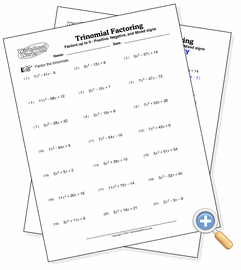# Trinomial Factoring

## Prime Variable CoefficientsDevelop trinomial factoring skills involving one coefficient

These are basic trinomial factoring problems where one of the second degree term has a prime number coefficient. This coefficient makes the problem more difficult by increasing the number of potential combinations needed to find the factors. However, there is still only one solution to each problem.